# Color Image Processing¶

Important: Please read the installation page for details about how to install the toolboxes. $\newcommand{\dotp}{\langle #1, #2 \rangle}$ $\newcommand{\enscond}{\lbrace #1, #2 \rbrace}$ $\newcommand{\pd}{ \frac{ \partial #1}{\partial #2} }$ $\newcommand{\umin}{\underset{#1}{\min}\;}$ $\newcommand{\umax}{\underset{#1}{\max}\;}$ $\newcommand{\umin}{\underset{#1}{\min}\;}$ $\newcommand{\uargmin}{\underset{#1}{argmin}\;}$ $\newcommand{\norm}{\|#1\|}$ $\newcommand{\abs}{\left|#1\right|}$ $\newcommand{\choice}{ \left\{ \begin{array}{l} #1 \end{array} \right. }$ $\newcommand{\pa}{\left(#1\right)}$ $\newcommand{\diag}{{diag}\left( #1 \right)}$ $\newcommand{\qandq}{\quad\text{and}\quad}$ $\newcommand{\qwhereq}{\quad\text{where}\quad}$ $\newcommand{\qifq}{ \quad \text{if} \quad }$ $\newcommand{\qarrq}{ \quad \Longrightarrow \quad }$ $\newcommand{\ZZ}{\mathbb{Z}}$ $\newcommand{\CC}{\mathbb{C}}$ $\newcommand{\RR}{\mathbb{R}}$ $\newcommand{\EE}{\mathbb{E}}$ $\newcommand{\Zz}{\mathcal{Z}}$ $\newcommand{\Ww}{\mathcal{W}}$ $\newcommand{\Vv}{\mathcal{V}}$ $\newcommand{\Nn}{\mathcal{N}}$ $\newcommand{\NN}{\mathcal{N}}$ $\newcommand{\Hh}{\mathcal{H}}$ $\newcommand{\Bb}{\mathcal{B}}$ $\newcommand{\Ee}{\mathcal{E}}$ $\newcommand{\Cc}{\mathcal{C}}$ $\newcommand{\Gg}{\mathcal{G}}$ $\newcommand{\Ss}{\mathcal{S}}$ $\newcommand{\Pp}{\mathcal{P}}$ $\newcommand{\Ff}{\mathcal{F}}$ $\newcommand{\Xx}{\mathcal{X}}$ $\newcommand{\Mm}{\mathcal{M}}$ $\newcommand{\Ii}{\mathcal{I}}$ $\newcommand{\Dd}{\mathcal{D}}$ $\newcommand{\Ll}{\mathcal{L}}$ $\newcommand{\Tt}{\mathcal{T}}$ $\newcommand{\si}{\sigma}$ $\newcommand{\al}{\alpha}$ $\newcommand{\la}{\lambda}$ $\newcommand{\ga}{\gamma}$ $\newcommand{\Ga}{\Gamma}$ $\newcommand{\La}{\Lambda}$ $\newcommand{\si}{\sigma}$ $\newcommand{\Si}{\Sigma}$ $\newcommand{\be}{\beta}$ $\newcommand{\de}{\delta}$ $\newcommand{\De}{\Delta}$ $\newcommand{\phi}{\varphi}$ $\newcommand{\th}{\theta}$ $\newcommand{\om}{\omega}$ $\newcommand{\Om}{\Omega}$

This numerical tour explores color image processing.

In :
addpath('toolbox_signal')


## RGB Color Space¶

A color image $f \in \RR^{N \times 3}$ is made of three independent images, one for each channel red, green and blue (RGB color space).

Size $N= n \times n$ of the image.

In :
n = 256;
N = n*n;


Loading an image $f \in \RR^{N \times 3}$.

In :
name = 'hibiscus';


One can display on screen a color image in RGB space using the rule of additive color mixing.

Display the image $f$ and the three channels that compose the colors.

In :
R = cat(3, f(:,:,1), zeros(n), zeros(n));
G = cat(3, zeros(n), f(:,:,2), zeros(n));
B = cat(3, zeros(n), zeros(n), f(:,:,3));
clf;
imageplot({f R G B}, ...
{ 'f' 'R (Red)' 'G (green)' 'B (blue)'}, 2, 2);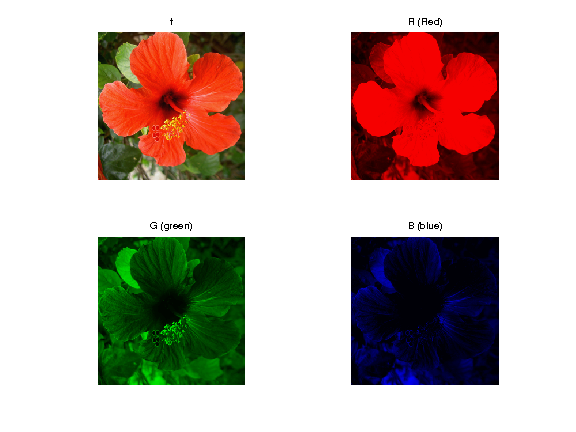It is possible to obtain a grayscale image from a color image by linear averaging of the channels, to obtain the luminance channel $$L = \frac{R+G+B}{3}$$

In :
clf;
imageplot({f mean(f,3)}, {'f' 'L'});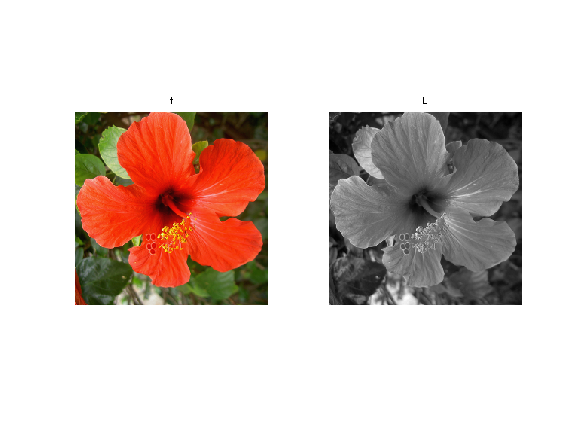## CMY Color Space¶

Another popular representation for color images uses as basis colors the cyan, magenta and yellow (CMY color space). They are computed as $$C=1-R, \quad f=1-G, \quad Y=1-B.$$

One can display on screen a color image in CMY space using the rule of substractive color mixing.

Show the C, f, Y channels.

In :
f1 = cat(3, f(:,:,1),     f(:,:,2)*0+1, f(:,:,3)*0+1);
f2 = cat(3, f(:,:,1)*0+1, f(:,:,2)    , f(:,:,3)*0+1);
f3 = cat(3, f(:,:,1)*0+1, f(:,:,2)*0+1, f(:,:,3));
clf;
imageplot({f f1 f2 f3}, ...
{ 'f' 'C' 'f' 'Y'}, 2, 2);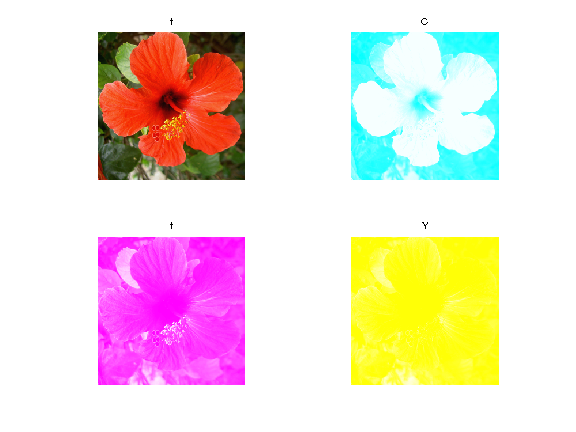## YUV Color Space¶

A linear color space is defined by applying a transformation matrix $T \in \RR^3$ to the RGB color channels of the image.

It means that each pixel values $f(x) \in \RR^3$ is replaced by $\tilde f(x) = T f(x)$, which store the three channels in the novel color space.

An example of transformation is the YUV color space, where Y is the luminance and UV are the chrominance. It is defined by $$T = \begin{pmatrix} 0.299 & 0.587 & 0.114 \\ -0.147 & -0.289 & 0.436 \\ 0.615 & -0.515 & -0.100 \end{pmatrix}.$$

In :
T = [.299 .587 .114; ...
-.14713 -.28886 .436; ...
.615 -.51499 -.10001]';


The RGB to YUV conversion is obtained by applying the matrix.

In :
applymat = @(f,T)reshape( reshape(f, [n*n 3])*T, [n n 3] );
rgb2yuv  = @(f)applymat(f,T);


Display the YUV channels.

In :
U = rgb2yuv(f);
clf;
imageplot(U(:,:,1), 'Y', 1,3,1);
imageplot(U(:,:,2), 'U', 1,3,2);
imageplot(U(:,:,3), 'V', 1,3,3);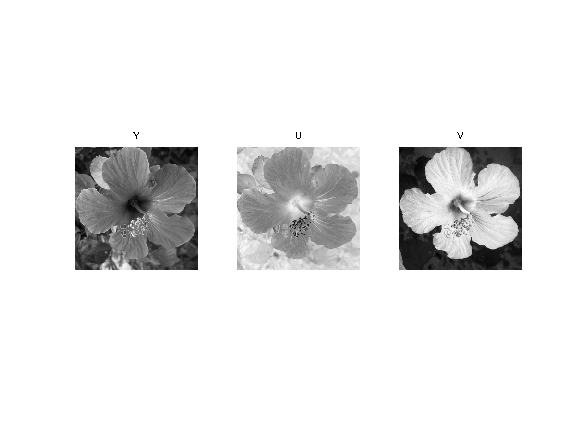The image can be modified by modifying the YUV representation $U$ to obtain $U_1$. Here we lower the chrominance of the image.

In :
U1 = U;
U1(:,:,2:3) = U1(:,:,2:3)/2;


Exercise 1

Recover an image from the transformed YUV representation $U_1$.

In :
exo1()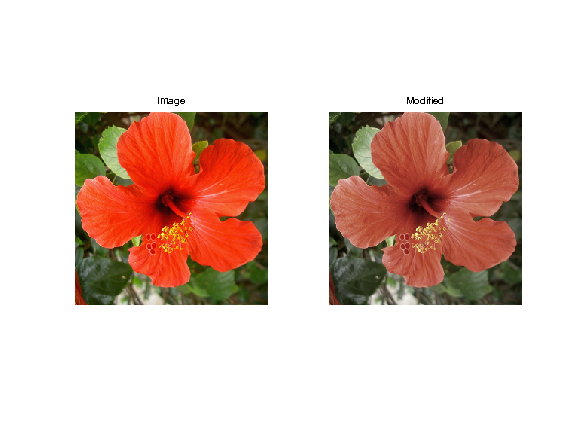In :
%% Insert your code here.


## HSV Color Space¶

A non-linear color space is obtained by a polar or conical parameterization of a linear color space. The angular coordinate in the plane orthogonal to the first linear axis (which is usually the luminance) is called the Hue, and the radial coordinates is called the saturation.

Using a luminance which is the sum of the 3 coordinates, one obtain a color system that is quite close to the HSV color system (which has a more complicated definition, but leads to similar results).

First we compute the value (luminance) coordinate, which is the orthogonal projection on $[1, 1, 1]$.

In :
Value = @(f)sum(f, 3) / sqrt(3);


The we compute the projection on the plane orthogonal to $[1, 1, 1]$, for instance using the projections $A$ and $B$ on the two orthognoal unit vectors $$[0, 1, -1]/\sqrt{2} \qandq [2, -1, -1]/\sqrt{6}.$$

In :
A = @(f)( f(:,:,2)-f(:,:,3) )/sqrt(2);
B = @(f)( 2*f(:,:,1) - f(:,:,2) - f(:,:,3) )/sqrt(6);


The $(V,A,B)$ components are obtained from RGB using a transformation with an orthogonal matrix $T$.

In :
T = [   1/sqrt(3) 1/sqrt(3) 1/sqrt(3); ...
0 1/sqrt(2) -1/sqrt(2); ...
2/sqrt(6) -1/sqrt(6) -1/sqrt(6)];


The Hue/Saturation are the polor coordinates within this plane.

In :
Saturation = @(f)sqrt( A(f).^2 + B(f).^2 );
Hue = @(f)atan2(B(f),A(f));


Shortcut for HSV color transformation. We name it |rgb2hsv1| because it is not exactly a mapping to the classical HSV space.

In :
rgb2hsv1 = @(f)cat(3, Hue(f), Saturation(f), Value(f));


Compute the transformation.

In :
g = rgb2hsv1(f);


Display.

In :
clf;
imageplot({g(:,:,1) g(:,:,2) g(:,:,3)}, {'H' 'S' 'V'}, 1,3);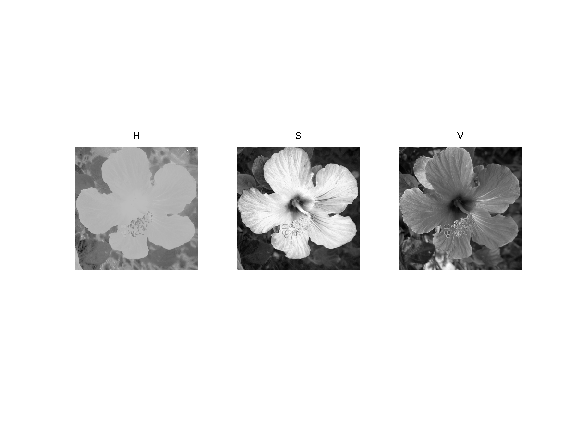Exercise 2

Show the reconstructed image obtained by progressively rotating the hue component, i.e. replacing $H$ by $H + \theta$. his ugly code is for Scilab compatibility

In :
exo2()